## A Particle in a Box

As a concrete illustration of these ideas, we study the particle in a box (in one dimension). This is just a particle (of mass) which is free to move inside the walls of a box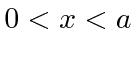, but which cannot penetrate the walls. We represent that by a potential which is zero inside the box and infinite outside. We solve the Schrödinger equation inside the box and realize that the probability for the particle to be outside the box, and hence the wavefunction there, must be zero. Since there is no potential inside, the Schrödinger equation is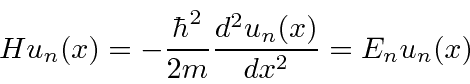where we have anticipated that there will be many solutions indexed by. We know four (only 2 linearly independent) functions which have a second derivative which is a constant times the same function: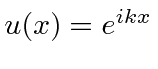,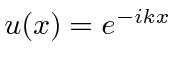,, and. The wave function must be continuous though, so we require the boundary conditions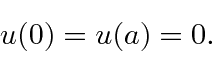The sine function is always zero atand none of the others are. To make the sine function zero at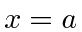we needor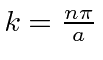. So the energy eigenfunctions are given bywhere we allow the overall constant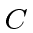because it satisfies the differential equation. Pluggingback into the Schrödinger equation, we find thatOnly quantized energies are allowed when we solve this bound state problem. We have one remaining task. The eigenstates should be normalized to represent one particle.So the wave function will be normalized if we choose.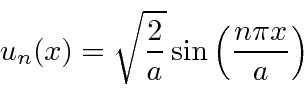We can always multiply by any complex number of magnitude 1, but, it doesn't change the physics. This example shows many of the features we will see for other bound state problems. The one difference is that, because of an infinite change in the potential at the walls of the box, we did not need to keep the first derivative of the wavefunction continuous. In all other problems, we will have to pay more attention to this.

Jim Branson 2013-04-22